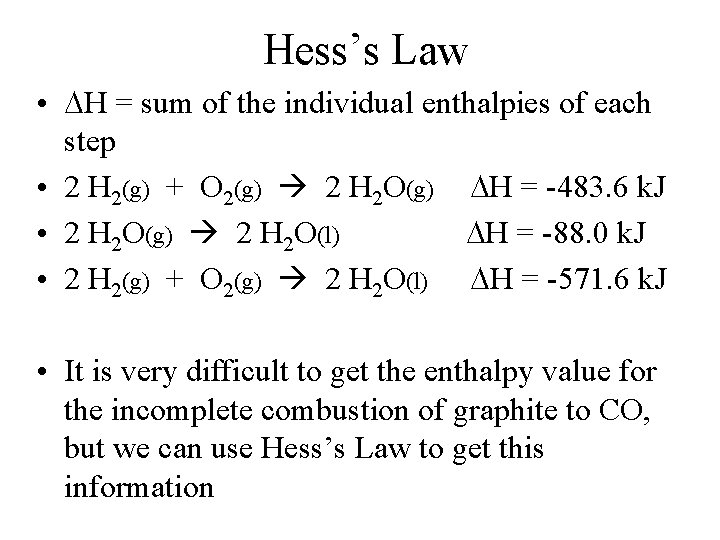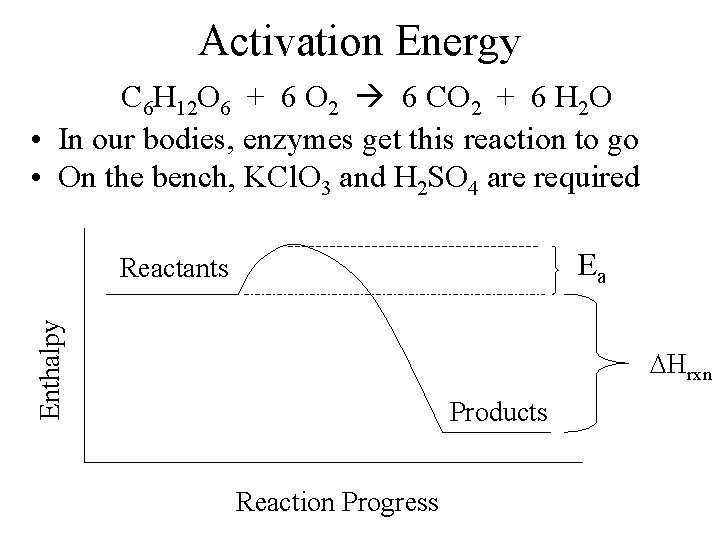# Chapter 4 CIC and Chapter 5 CTCS Read

• Slides: 6
Download presentationChapter 4 (CIC) and Chapter 5 (CTCS) • Read in CTCS Chapter 5. 6, 7, pgs 172 -3 • Problems in CTCS: 49, 51, 53, 57, 59, 61, 63, 65, 67, 75Hess’s Law • H = sum of the individual enthalpies of each step • 2 H 2(g) + O 2(g) 2 H 2 O(g) H = -483. 6 k. J • 2 H 2 O(g) 2 H 2 O(l) H = -88. 0 k. J • 2 H 2(g) + O 2(g) 2 H 2 O(l) H = -571. 6 k. J • It is very difficult to get the enthalpy value for the incomplete combustion of graphite to CO, but we can use Hess’s Law to get this informationC(g) + O 2(g) CO 2(g) H = -393. 5 k. J CO(g) + 1/2 O 2(g) CO 2(g) H = -283. 0 k. J Q: What is Hºf of NO 3 -(aq)? Hint: HNO 3 is a strong acid A: -206. 6 k. JActivation Energy C 6 H 12 O 6 + 6 O 2 6 CO 2 + 6 H 2 O • In our bodies, enzymes get this reaction to go • On the bench, KCl. O 3 and H 2 SO 4 are required Ea Enthalpy Reactants Hrxn Products Reaction ProgressPhotosynthesis • Ultimate source of all fossil fuels is the sun • 6 CO 2 + 6 H 2 O C 6 H 12 O 6 + 6 O 2 ΔH = +2800 k. J • Catalyst is chlorophyll • Wood • Coal – Solid – Little Oxygen but fair amount of Sulfur • Petroleum – Liquid – Less Oxygen and Sulfur – Must be refinedReformulated Gasolines (RFG’s) • Get rid of Pb. Et 4 • Must be 2% by mass oxygen • Use MTBE or Et. OH Q: If MTBE is C 5 H 12 O and gasoline is C 8 H 18, What percentage of MTBE must be in RFG? A: 14%